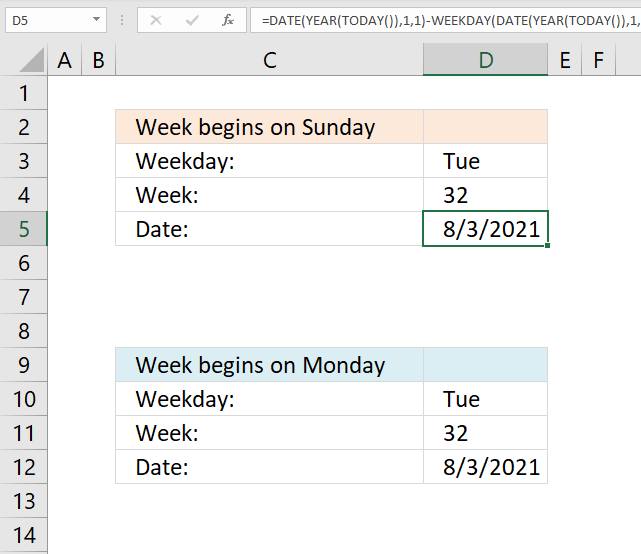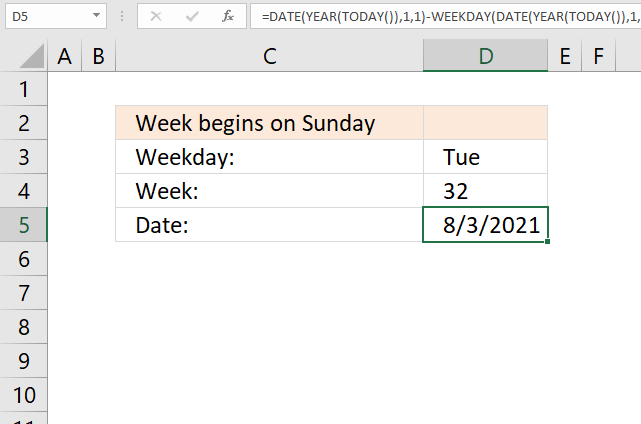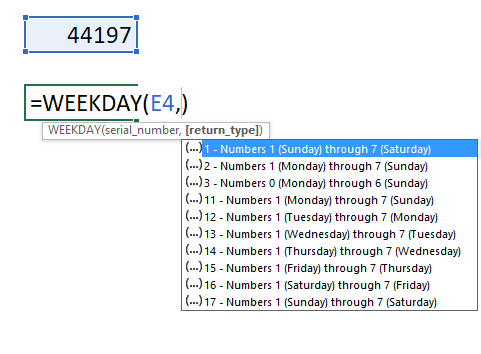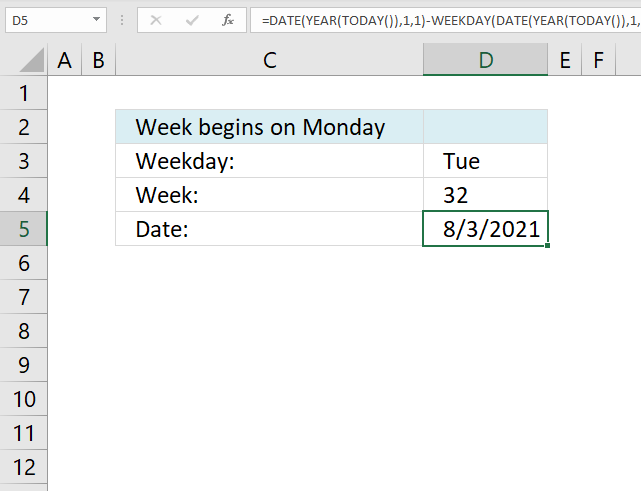Author: Oscar Cronquist Article last updated on February 10, 2023This article demonstrates a formula that returns a date based on a week number and a weekday like Sun to Sat. It uses the current year to calculate the date.

I want to find the day if given date and week, for example: if given Tuesday, 32nd week, 2015 >>> how to create a formula to point out 4/8/2015?

## 1. Calculate date given weekday and week number (week starts on Sunday)Formula in cell D6:

=DATE(YEAR(TODAY()), 1, 1)-WEEKDAY(DATE(YEAR(TODAY()), 1, 1), 1)+(7*(D4-1))+MATCH(\$D\$4, {"Sun", "Mon", "Tue", "Wed", "Thu", "Fri", "Sat"}, 0)

### Explaining formula in cell D6

#### Step 1 - Calculate the first date of this year

The TODAY function returns today's date, the function is volatile meaning it recalculates every time you enter a value in a cell and press enter or press F9.

TODAY() returns 5/8/21.

#### Step 2 - Calculate the first date of this year

The YEAR function returns the year based on an Excel date.

YEAR(TODAY())

becomes

YEAR(44324)

and returns 2021.

#### Step 3  - Calculate the first date of this year

The DATE function returns an Excel date based on a year number, month number and day number.

DATE(year, month, day)

Year is a number from 1900 to 9999, month is a number from 1 to 12. 1 = January, ... , 12 = December. Day is usually a number between 1 and 31 but can be larger.

DATE(YEAR(TODAY()), 1, 1)

becomes

DATE(2021, 1, 1)

and returns 44197.

#### Step 4 - Subtract with the corresponding weekday number

The WEEKDAY function converts an Excel date to a number ranging from 1 to 7, based on when the week begins.

WEEKDAY(serial_number, [return_type])If you live in the US the week in starts on Sundays, return_type is 1.

WEEKDAY(DATE(YEAR(TODAY()), 1, 1), 1)

becomes WEEKDAY(44197, 1)

and returns 6. 44197 is 1/1/2021 and that day is a Friday.

1 - Sunday, ... , 7 - Saturday.

#### Step 5 - Subtract date with weekday number

DATE(YEAR(TODAY()), 1, 1)-WEEKDAY(DATE(YEAR(TODAY()), 1, 1), 1)

becomes

44197 - 6 equals 44191.

#### Step 6 - Calculate and add the number of days from Monday/week 1 to Monday/(nth week -1)

+7*(D4-1)

Example, 7*(32-1)

becomes

7*31 and returns 217.

#### Step 7 - Calculate and add weekday number

The MATCH function returns the relative position of the given item in a cell range or array.

MATCH(\$D\$4, {"Sun", "Mon", "Tue", "Wed", "Thu", "Fri", "Sat"}, 0)

If you week begins on sunday, this is the array you want to be using:

{"Sun", "Mon", "Tue", "Wed", "Thu", "Fri", "Sat"}.

Example, "Tue" returns 3. "Tue" is in position three in the array.

#### Step 8 - Add and subtract

44192+217+2 = 44411

44411 is 8/3/2021.

## 2. Calculate date given weekday and week number (week starts on Monday)This example demonstrates a formula that works with weeks that begin with Mondays.

Formula in cell 5:

=DATE(YEAR(TODAY()), 1, 1)-WEEKDAY(DATE(YEAR(TODAY()), 1, 1), 2)+(7*(D4-1))+MATCH(\$D\$3, {"Mon", "Tue", "Wed", "Thu", "Fri", "Sat", "Sun"}, 0)

Note that the WEEKDAY function uses 2 in the second argument meaning the week begins with a Monday and the array in the second argument in the MATCH function begins with Monday.